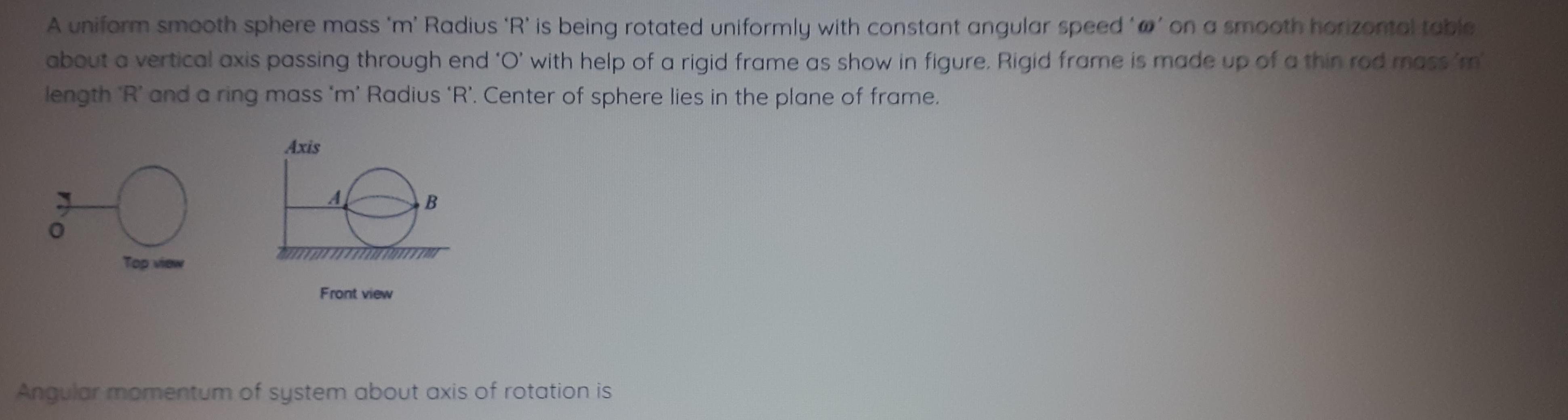Question:

# A uniform smooth sphere mass 'm' Radius 'R' is being rotatedA uniform smooth sphere mass 'm' Radius 'R' is being rotated uniformly with constant angular speed 'w' on a smooth horizontal table about a vertical axis passing through end 'O' with help of a rigid frame as show in figure. Rigid frame is made up of a thin rod mass 'm length 'R' and a ring mass 'm' Radius 'R'. Center of sphere lies in the plane of frame. Angular momentum of system about axis of rotation is